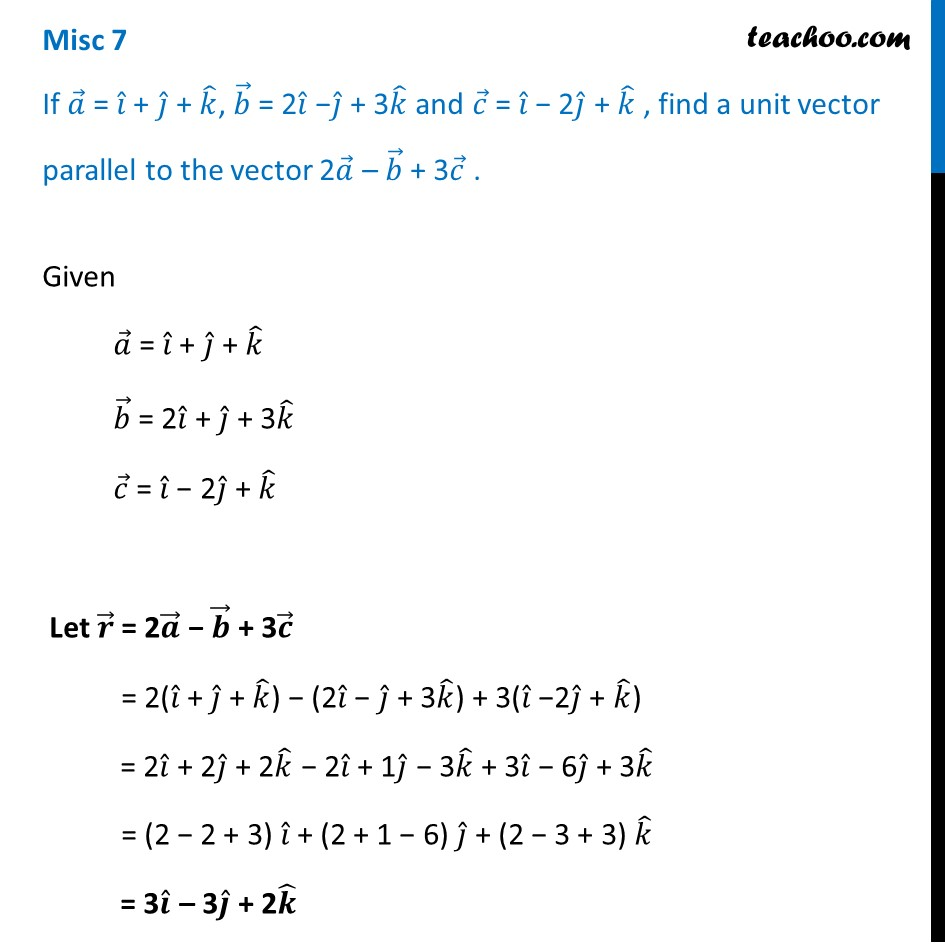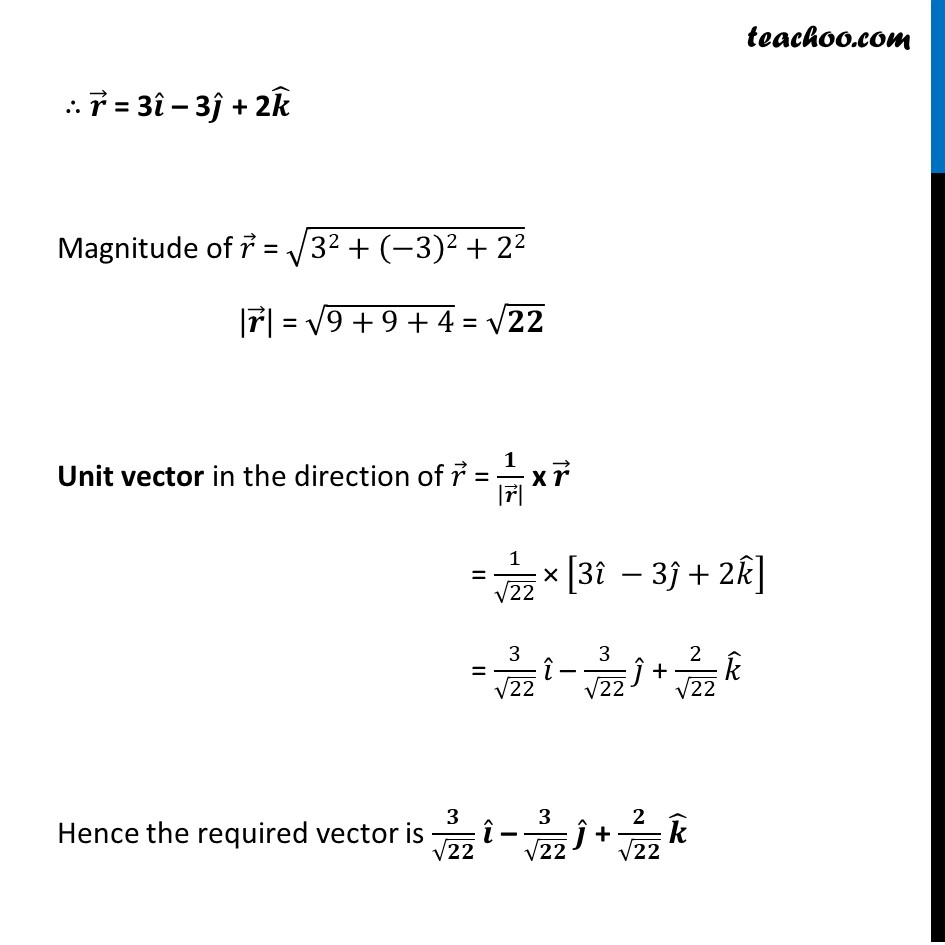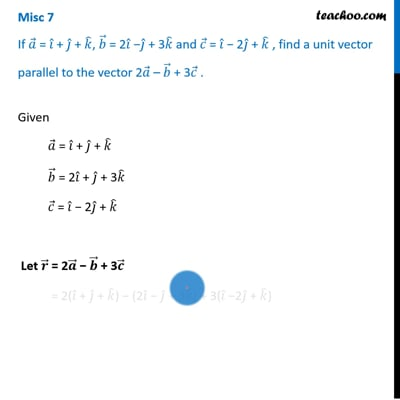Chapter 10 Class 12 Vector Algebra
Concept wiseThis video is only available for Teachoo black users

Introducing your new favourite teacher - Teachoo Black, at only ₹83 per month

### Transcript

Misc 7 If 𝑎 ⃗ = 𝑖 ̂ + 𝑗 ̂ + 𝑘 ̂, 𝑏 ⃗ = 2𝑖 ̂ −𝑗 ̂ + 3𝑘 ̂ and 𝑐 ⃗ = 𝑖 ̂ − 2𝑗 ̂ + 𝑘 ̂ , find a unit vector parallel to the vector 2𝑎 ⃗ – 𝑏 ⃗ + 3𝑐 ⃗ . Given 𝑎 ⃗ = 𝑖 ̂ + 𝑗 ̂ + 𝑘 ̂ 𝑏 ⃗ = 2𝑖 ̂ + 𝑗 ̂ + 3𝑘 ̂ 𝑐 ⃗ = 𝑖 ̂ − 2𝑗 ̂ + 𝑘 ̂ Let 𝒓 ⃗ = 2𝒂 ⃗ − 𝒃 ⃗ + 3𝒄 ⃗ = 2(𝑖 ̂ + 𝑗 ̂ + 𝑘 ̂) − (2𝑖 ̂ − 𝑗 ̂ + 3𝑘 ̂) + 3(𝑖 ̂ −2𝑗 ̂ + 𝑘 ̂) = 2𝑖 ̂ + 2𝑗 ̂ + 2𝑘 ̂ − 2𝑖 ̂ + 1𝑗 ̂ − 3𝑘 ̂ + 3𝑖 ̂ − 6𝑗 ̂ + 3𝑘 ̂ = (2 − 2 + 3) 𝑖 ̂ + (2 + 1 − 6) 𝑗 ̂ + (2 − 3 + 3) 𝑘 ̂ = 3𝒊 ̂ – 3𝒋 ̂ + 2𝒌 ̂ ∴ 𝒓 ⃗ = 3𝒊 ̂ – 3𝒋 ̂ + 2𝒌 ̂ Magnitude of 𝑟 ⃗ = √(32+(−3)2+22) |𝒓 ⃗ | = √(9+9+4) = √𝟐𝟐 Unit vector in the direction of 𝑟 ⃗ = 𝟏/|𝒓 ⃗ | x 𝒓 ⃗ = 1/√22 × [3𝑖 ̂ −3𝑗 ̂+2𝑘 ̂ ] = 3/√22 𝑖 ̂ – 3/√22 𝑗 ̂ + 2/√22 𝑘 ̂ Hence the required vector is 𝟑/√𝟐𝟐 𝒊 ̂ – 𝟑/√𝟐𝟐 𝒋 ̂ + 𝟐/√𝟐𝟐 𝒌 ̂